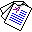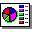# Constructing Consensus Logic Programs

## Chiaki Sakama and Katsumi Inoue

Proceedings of the 16th International Symposium on Logic-based Program Synthesis and Transformation (LOPSTR'06), Lecture Notes in Computer Science 4407, Springer-Verlag, pages 26-42, 2007.

## Abstract

In this paper, we suppose an agent which has a knowledge base represented by a logic program under the answer set semantics. We then consider the following two problems: given two programs P1 and P2, which have the sets of answer sets AS(P1) and AS(P2), respectively; (i) find a program Q which has the answer sets as the minimal elements of { S ∩ T | S ∈ AS(P1) and T ∈ AS(P2) }; (ii) find a program R which has the answer sets as the maximal elements of the above set. A program Q satisfying (i) is called minimal consensus between P1 and P2; and R satisfying (ii) is called maximal consensus between P1 and P2. Minimal/maximal consensus extracts common beliefs that are included in an answer set of every program. Consensus provides a method of program development under a specification of constructing a program that reflects the meaning of two or more programs. In application, it contributes to a theory of building consensus in multi-agent systems.Full Paper (PDF 375K)Slide (pdf 124K) , © Springer-Verlag